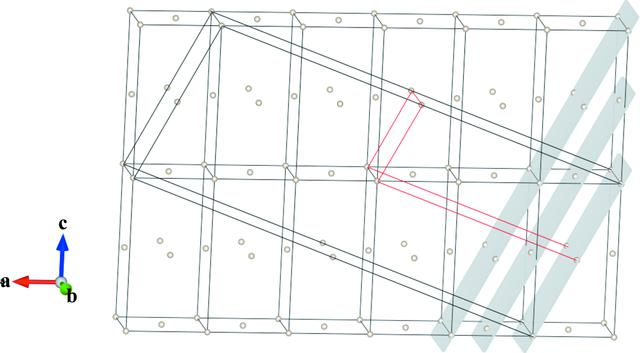disable zoom     view article Figure 1 Twelve unit cells of sulfur, of type oF, in the intervals u = [−1, 5], v = [0, 1], w = [0, 2]. The unit cell in black is obtained by the transformation a′ = 5a + c; b′ = b; c′ = −a + c, based on the incorrectly simplified Miller and direction indices of the lattice elements (plane, direction) defining the twin lattice, namely (101) and . This unit cell is B centred and has a volume six times larger that the unit cell of the individual crystal, which would suggest a twin index n = 6 × 4/2 = 12, but actually contains lattice nodes also at, 0, 0 and 0, 0,. The real unit cell of the twin lattice is shown in red and is obtained by the transformation a′ = 5a/2 + c/2; b′= b; c′ = −a/2 + c/2, based on the correctly expressed Miller and direction indices of the lattice elements defining the twin lattice, namely (202) and. This unit cell is I centred and is only 1.5 times bigger than the unit cell of the individual crystal, giving the correct twin index n = 1.5 × 4/2 = 3 (figure drawn with VESTA: Momma & Izumi, 2011).JOURNAL OFAPPLIEDCRYSTALLOGRAPHY
ISSN: 1600-5767
Volume 50| Part 5| October 2017| Pages 1541-1544